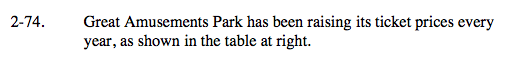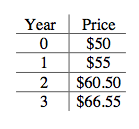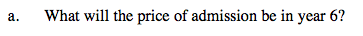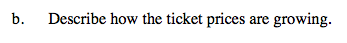### Home > CAAC > Chapter 14 > Lesson 14.2.1.5 > Problem2-74

2-74.The price is multiplied by 1.1 each year.

Year 4 = $66.55 · 1.1 =$73.205
Year 5 = $73.205 · 1.1 =$80.5255
Year 6 = $80.5255 · 1.1 =$88.57805 ≈ $88.58 The price will be$88.58 in year 6.How did you find the price for each subsequent year in part (a)?